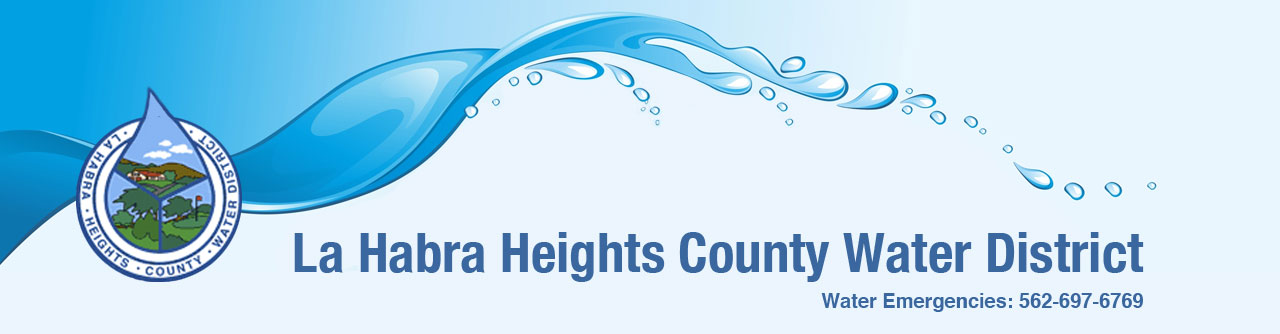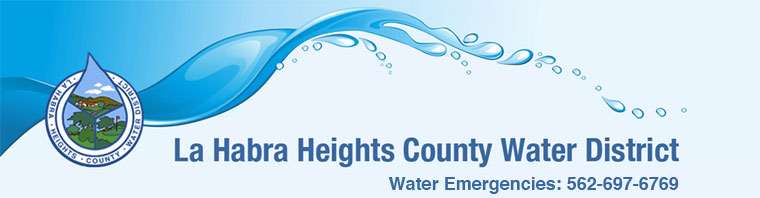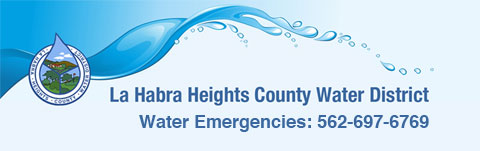# La Habra Heights County Water District## Filling a Swimming Pool

If you are planning maintenance on your swimming pool and it requires draining the water you may want to know several things. First, there is no need to contact the District to let us know that you are draining the water. Second, you may want to know how much it cost to fill your pool after it has been drained. To calculate the amount of water it will take to fill your pool you will need the dimensions (depth, width, length) of your pool in feet.

Example:
Pool dimension: Depth = 5 feet, Width = 20 feet, Length = 30 feet

Multiply (depth x width x length): 5 x 20 x 30 = 3,000 cubic feet Divide by 100 resulting in units of water usage: 3,000 / 100 = 30 hundred cubic feet. Then multiply the hundred cubic feet usage by the water usage charge (see below). One Hundred cubic feet of water is equal to one unit of water usage. If you live in the upper zone, the total water usage charge rate is 2.98 per usage; lower zone rate is 2.78

Estimated water cost to fill pool: 30 hundred cubic feet x 2.98 = \$89.40